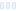# Superfood Formula, Original

## True Moo

Main info:

Superfood Formula, Original
True Moo
8 1/2 g
35 Calories
4 g
1 g
2 g

2 g
0 mg
0 g
20.0 mg
1 g
0 g

Nutrition Facts
For a Serving Size of (g)
How many calories are in Superfood Formula, Original? Amount of calories in Superfood Formula, Original: Calories Calories from Fat (%)
% Daily Value *
How much fat is in Superfood Formula, Original? Amount of fat in Superfood Formula, Original: Total Fat
How much sodium is in Superfood Formula, Original? Amount of sodium in Superfood Formula, Original: Sodium
How many carbs are in Superfood Formula, Original? Amount of carbs in Superfood Formula, Original: Carbohydrates
How many net carbs are in Superfood Formula, Original? Amount of net carbs in Superfood Formula, Original: Net carbs
How much sugar is in Superfood Formula, Original? Amount of sugar in Superfood Formula, Original: Sugar
How much fiber is in Superfood Formula, Original? Amount of fiber in Superfood Formula, Original: Fiber
How much protein is in Superfood Formula, Original? Amount of protein in Superfood Formula, Original: Protein
Vitamins and minerals
How much Vitamin A is in Superfood Formula, Original? Amount of Vitamin A in Superfood Formula, Original: Vitamin A
How much Vitamin C is in Superfood Formula, Original? Amount of Vitamin C in Superfood Formula, Original: Vitamin C
How much Calcium is in Superfood Formula, Original? Amount of Calcium in Superfood Formula, Original: Calcium
How much Iron is in Superfood Formula, Original? Amount of Iron in Superfood Formula, Original: Iron
Fatty acids
Amino acids
* The Percent Daily Values are based on a 2,000 calorie diet, so your values may change depending on your calorie needs.
Percent calories from...Loading similar foods...
Note: Any items purchased after clicking our Amazon buttons will give us a little referral bonus. If you do click them, thank you!Be cool

- Zen orangeI never skip arm day

- Buff broccoli